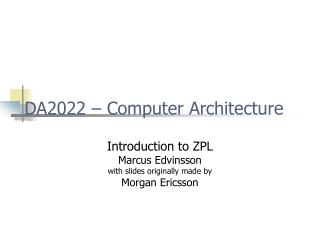DownloadDownload PresentationDA2022 – Computer Architecture

# DA2022 – Computer Architecture

Télécharger la présentation## DA2022 – Computer Architecture

- - - - - - - - - - - - - - - - - - - - - - - - - - - E N D - - - - - - - - - - - - - - - - - - - - - - - - - - -
##### Presentation Transcript

1. DA2022 – Computer Architecture Introduction to ZPL Marcus Edvinsson with slides originally made by Morgan Ericsson

2. Today • Introduction to the assignments • ”The deal” • The assignment • Introduction to ZPL • What is ZPL • Getting started • ZPL and lots of examples

3. ”The deal” • Everything you need is on the course page or linked to from the course page, except • /stud/kurser/da2022 • I introduce the assignment and the different techniques and environments and I also answer your questions and correct/grade your (practical) work/assignments

4. The assignments • Parallel programming • ZPL (today) • MPI (later) • Image manipulation • Grey-scale conversion • Histogram • Smoothing

5. PPM and PGM • Format used for images in this course • You should be able to load/save these formats • Very simple formats • ASCII readable (mostly) • Raw and ASCII (we like it raw!) • Uncompressed • Lots of available tools • NetPBM (/usr/local/netpbm) • XV (/usr/local/bin) • Etc (google!)

6. PPM and PGM • Format [MAGIC] # Optional comment [WIDTH] [HEIGHT] [MAXIMUM COLOR COMPONENT VALUE] Image data (RGB) * HEIGHT * WIDTH • Height, width, etc, ”ASCII formatted” integers, e.g., 640 • Magic identifies the format, P5 PGM RAW, P6 PPM RAW

7. Example P6 # CREATOR: XV Version 3.10a Rev: 12/29/94 720 480 255 ^O^M^N^P^N^O^Q^Q^Q^Q^S^R^M^O^N ^M^L^N^T ^R^X^^ ^\ ^^\$" &\$#'&#'&%%%%%%&\$%&&&%%'\$%'\$%'#\$&#\$&"#%”#%"#% #\$&\$%'\$%'%&(%&(\$%'\$%'#\$&"\$##%\$#%\$\$&%\$&%#%\$#%\$"\$#! #"!#""\$##%\$#%\$"\$#!#"!#""#%"#%"#%"#%"#%"#%"#%"#%#\$ &\$%'\$%'\$%'\$%'#\$&"#%!"\$#%\$#%\$#%\$\$&%\$&%%'&%'&%'&#%\$ #%\$#%\$\$&%\$&%%'&%'&%'& …

8. ZPL • High-level, platform independent • Implicitly parallel • Can be run in parallel or sequentially • Array programming language • Operations can be applied to arrays (of any dimension)

9. Getting started • ZPL is installed in the Unix lab rooms • Set the following environment variables: • Set ZPLHOME to /opt/zplhome • Set ZPLCOMMLAYER to seq • Set ZPLTARGET to sparc-solaris • (Syntax depending on shell, use setenv if csh) • Or • Run /stud/kurser/da2022/instMPI • Then what? • Compiler is zc • Executables are named like the source, but without .z

10. ZPL • Syntax very similar to Pascal (and Ada) • Please note • /* */ comments • := assignment, = comparison

11. Example 1 program Ex1; procedure Ex1(); begin writeln("Hello, world!"); end;

12. Example 2 program Ex2; var a,b:integer; procedure Ex2(); begin b:=10; a:=a+b; a+=b;; writeln("a=",a,"."); write("a=%0d.\n":a); write("a=%0d":a, ", b=%0d.\n":b); end;

13. Example 3 program Ex3; var a,b,i:integer; procedure Ex3(); begin a:=0; for i:=1 to 5 do a+=i; end; writeln(a); end;

14. Example 4 program Ex4; var a,b:integer; procedure Ex4(); begin read(a); read(b); if a<b then writeln("a<b"); elsif a>b then writeln("a>b"); else writeln("a=b"); end; end;

15. (Parallel) Arrays and Regions • Regions = indices • Used to declare arrays or specify ”regions” that operations should operate on • Example: • region R = [1..10]; • a: [R] integer; • [R] a:=1; • Not first-class objects!

16. Example 5 program Ex5; region R = [1..10]; var a: [R] integer; procedure Ex5(); begin [R] a:=1; [R] write(a); end;

17. Example 6 program Ex6; region R = [1..10]; R2 = [3..6]; var a,b: [R] integer; procedure Ex6(); begin [R] a:=1; [R] b:=Index1; [R2] a+=b; [R] write(a); end;

18. Directions • ”Position-independent” way of indexing arrays

19. Directions • Defined by giving rules for transforming an index direction north = [-1, 0]; • Parallel arrays can not be indexed in other ways

20. Region Operators • Used to index/create new regions based on regions and/or directions • Of, creates a new region relative to a region and a direction • At (@), ”indexing” (displacement) by direction (can be used with variables as well) • In, creates a new region inside another region

21. Example 7 program Ex7; region R = [1..10]; R2 = [3..6]; direction above = [+1]; below = [-1]; var a,b: [R] integer; procedure Ex7(); begin [R] a:=1; [R] b:=Index1; [R2] a+=b; [above of R2] a:=99; [below of R2] a:=-100; [R] write(a); end;

22. Scans and Reductions • Operations that calculates ”something” based on the whole array • Reductions reduces the array to a single value • Scans propagate the values through the array • A = [ 1, 3, 2 ] • max<<A = 3 (R) • max||A = [ 1, 3, 3 ] (S)

23. Reductions • Available reductions (scans similar) +<< *<< max<< min<< &<< (and) |<< (or)

24. Example 8 program Ex8; region R = [1..10]; var a,b: [R] integer; m,s: integer; procedure Ex8(); begin [R] a:=1; [R] b:=Index1; [R] m:=max<<b; [R] s:=+<<b; [R] a:=+||a; end;

25. Config variables • Can be used to set constants at runtime • Array sizes or regions, for example • executable –svariable=value • ./ex9 –sn=5

26. Example 9 program Ex9; config var n:integer = 10; region R = [1..n,1..n]; var a: [R] integer; m,s: integer; procedure Ex9(); begin [R] a:=Index1*Index2; [R] m:=max<<a; [R] s:=+<<a; end;

27. Records • Composite types, like records are available type Name = record Definitions … ; end; • ’.’is used to access values in a record (like Ada, C) myRecord.myInteger := 5; • (See example 11 for more information)

28. Now what? • Use the reference manual • Try to understand the examples • Not all available examples were used in this presentation • File I/O (ex11) • Experiment!